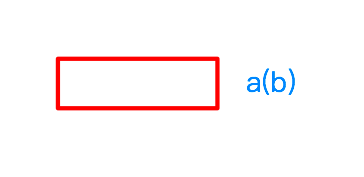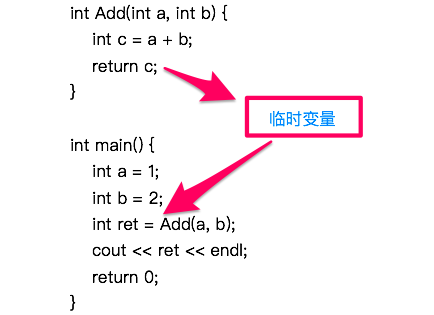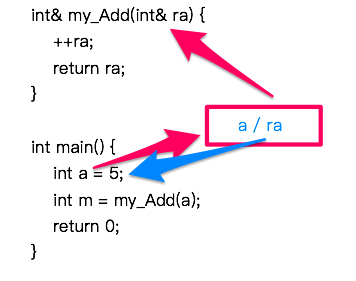# C++ | 引用变量

## 导语：为什么要有引用变量

void Swap(int* a, int* b) {
int tmp = *a;
*a = *b;
*b = tmp;
}

int main() {
int a = 5;
int b = 3;
Swap(&a, &b);
return 0;
}


## 引用的概念### 用法示例

int a;
int& b = a;


int main() {
int a = 5;
int& b = a;
cout << &a << endl; // 取a的地址
cout << &b << endl; // 取b的地址
return 0;
}


0x7fff5fbff80c
0x7fff5fbff80c

在这里， a 变量的地址是在他定义之时随机分配的，但 b 不是，他是根据自己即将要引用的变量的地址来分配。


int main(int argc, const char * argv[]) {
int a = 5;
int& b = a;

int c = 20;
b = c;
cout << a << endl; // 输出a修改后的值
cout << b << endl; // 输出b修改后的值
return 0;
}


20
20

### 引用的属性与特别之处

void test(int& ra) {
// ...
}

int main(int argc, const char * argv[]) {
int a = 5;
test(a + 3);
return 0;
}


1.实参类型正确，但不是左值
2.实参类型不正确，但可以转换为正确的类型

void test(int& ra) {
++ra;
}

int main(int argc, const char * argv[]) {
int a = 5;
double b = 4;

test(a + 3);
test(b);
return 0;
}


void test(const int& ra);


## 主要作用

### 1.引用作参数

#### 用法

void Swap(int& x, int& y) {
int tmp = x;
x = y;
y = tmp;
}

int main(int argc, const char * argv[]) {
int a = 5;
int b = 3;
Swap(a, b);
return 0;
}


int cube(int& ra) {
ra *= ra * ra;
return ra;
}

int main() {
int a = 3;
int ret = cube(a);
cout << "cube of " << a << " = "<< ret << endl;
return 0;
}


int cube(const int& ra);


#### 小结

1. 在您打算修改原变量的值时，尽量使用引用作参数，省时间省空间，更重要的是比采取指针传参的方式简单很多；
2. 在您并不打算改变变量的值，但是写了一个函数，要使用到您变量的值的时候，可以采用 const + 引用 的方式，表面上看和从前两者都不用的时候效果是一样的，但实际上省了很多的时间与空间（在传参较大时）。

### 2.引用作返回值

#### 为何要返回引用

double m = sqrt(16.0);a 自增以后再返回其值，利用引用，这时候并不需要考虑生命周期的问题，因为来来回回都是在堆那一块空间进行修改，一直在 a / ra 的作用域内，省去了再复制一步的时间和空间。

#### 返回引用时要注意的问题

int& Add(int a, int b) {
int c = a + b;
return c;
}

int main() {
cout << ret << endl;
return 0;
}


#### 小结

1. 引用作返回值，不能返回一个临时变量的引用，需要变量在这个函数结束后还在，例如静态或全局变量
2. 如果可以的话，我们尽量用引用作返回值，因为更省时间和空间

## 何时使用引用参数

### 1. 传递值而不修改值(尽量 const 修饰)

 1、内置数据类型：由于较小，可直接按值传递；
2、数组：采用 const 修饰的指针；
3、较大的结构：使用 const 指针或 const 引用，可提高效率、节省时间空间；
4、类对象：const 引用。


### 2. 需要修改原数据

 1、内置数据类型：可使用指针；
2、数组：只能使用指针；
3、较大的结构：使用指针或引用；
4、类对象：const 引用。


## 实现方法（汇编层来看）

1. 引用必须初始化，并且从一而终，但指针可以修改指向；
2. 引用必须指向有效变量，指针可以指向空；
3. 指针代表着地址，而引用代表着变量。sizeof 指针，是指针的大小，sizeof 变量，是变量的大小；
4. 引用与指针自增自减的意义不同。
5. 引用比指针更安全，没有野指针的危险，但同时指针也比引用灵活。